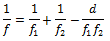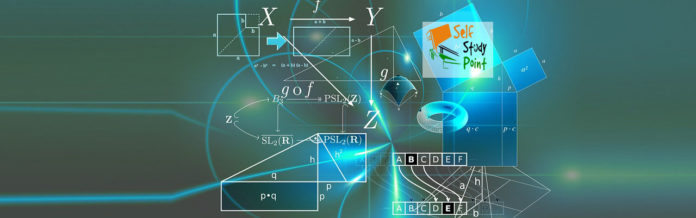Power of a lens is defined as the ability of the lens to converge a beam of light falling on the lens.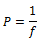If more than one lens are combined then total Power is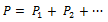Similarly,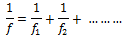If the lenses of focal lengths f1 and f2 are separated by a distance d then the combined focal length is